Analysis WebNotes
arrow_back arrow_forward

# Class Contents

## The Definition of the Riemann Integral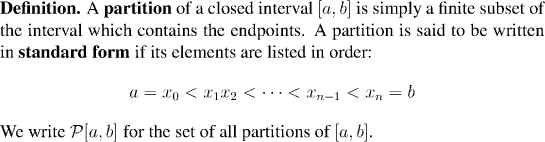Partitions are ordered by set inclusion. This isn't a total order (i.e. there can be two partitions, neither of which contains the other) but it does have two properties which will be very useful for our purposes. We shall point them out explicitly here, and even although they may seem totally obvious, they will be the key properties of partitions for most of what we need to do with them:
• The relation of set inclusion is transitive: if P is contained in Q and Q is contained in R then P is contained in R.
• Set inclusion is upward filtering: if P and Q are two partitions then there is a third partition R which contains both of them (this is just their union!). Thus, although there is no reason why P should contain Q or vice versa, at least we can find another partition to contain both.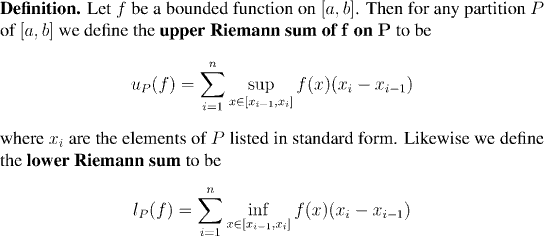Remark: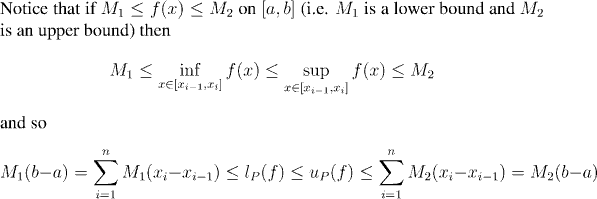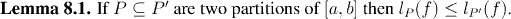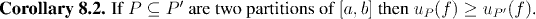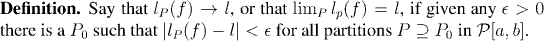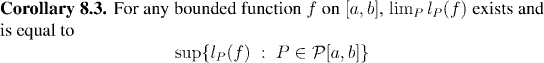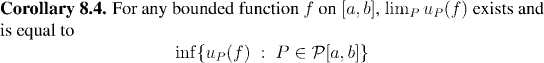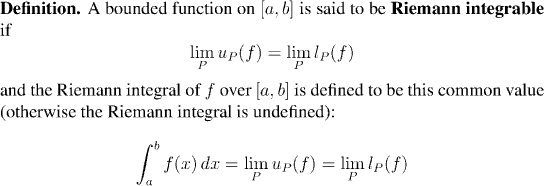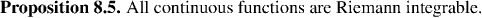Example: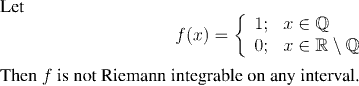Remark:
Just because a function is Riemann integrable, there is no reason to belive that the indefinite integral can be evaluated in analytic form (i.e. in terms of polynomials, exponentials). All it means is that the limits that we use to evaluate the integral give unambiguous results. Plenty of functions for which the indefinite integral cannot be expressed in closed form are nevertheless integrable.
Remark:
Note also that the definition we have here is for Riemann integrability. There are other definitions of the integral, which have their own (typically larger) families of integrable functions. (The Lebesgue integral is the most important example.)